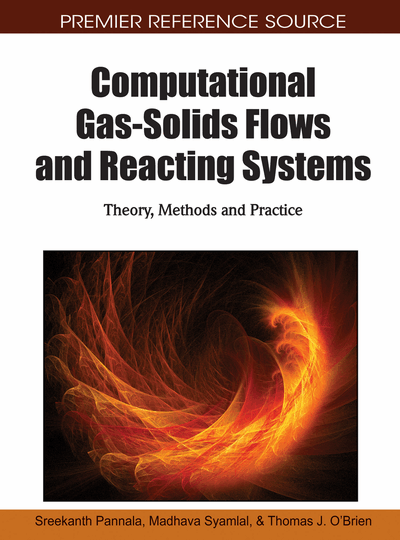# Mass and Heat Transfer Modeling

Ronald W. Breault (National Energy Technology Laboratory, USA)
DOI: 10.4018/978-1-61520-651-3.ch005
Available
\$37.50
No Current Special Offers

## Abstract

Mass and heat transfer are important to reactor modeling using CFD. Reactants (mass) move within the flow structure in order to react with other species and thereby form the products, both desirable and undesirable. This movement in mass occurs either by diffusion or by turbulent dispersion. In a similar fashion, heat is transferred from one point in the flow structure to another point by convective transfer between the phases and with the translational effect that occurs with the turbulent dispersion of the mass. In addition, heat can be transferred across the system boundary. In all these cases, fundamental mechanistic models are put forward that can be incorporated in the CFD code to calculate these transfer properties based upon the local hydrodynamic conditions. The chapter is organized such that dense phase systems are covered first and then dilute phase systems. Within each of these areas mass transfer is covered first and followed by heat transfer. The topics are covered in the following order: Diffusional mass transfer, Turbulent dispersion, Convective heat transfer between phases and Convective heat transfer at the system boundary.
Chapter Preview
Top

## Introduction

Prior to jumping into the discussion of the diffusional mass transfer, heat transfer and the turbulent dispersion, a short overview of the balances contained within MFIX is shown below such that these properties can be identified. Interphase mass transfer occurs by two mechanisms in heterogeneous gas-solids flows. The first of these is through diffusion across the boundary layer and the other is from turbulent transport of the species in gas eddies or solids clusters. Before to getting into the details of these two mechanisms and the methods which will be used to calculate these quantities, it is important to understand how and where these properties are used in reacting computational multiphase flow fluid dynamics.

For isothermal conditions, the continuity, momentum, and species balance equations are given below.

Gas-Phase Continuity

(1)

Solids-Phase Continuity

(2)

Gas-Phase Momentum

(3)

Solids-Phase Momentum

(4)

Species Balance

(5) where m = g or s for the gas or solids phase, Dmn is the turbulent diffusive term, Rmn is the reaction rate which is dependent upon the diffusional mass transfer, and ξ̅0 = 1 − ξ0, ξ0 = 1 if R0< 0 else ξ0 = 0. The eight dependent hydrodynamic variables in three dimensions are void fraction εg (the solids fraction εs = 1 − εg), pressure Pg, and six velocity components. These are found by using MFIX to numerically solve the coupled non-linear partial differential equations. The number of species mass fractions (Xmn) tracked are given in the chemistry model. Constitutive relations needed to close the system, and the gas/solids energy balance equations can be found in the following references (Syamlal, M., Rogers, W., & O’Brien, T. (1993); Syamlal, M.(1998)). A discussion on the solution procedure and further numerical references was prepared by Guenther & Syamlal (2001).

In general, the reaction rate or kinetics follow a conventional resistance model pathway, where the rate is is proportional to the sum of the resistance elements such as transfer from the bulk to the particle surface plus the internal resistance which may include diffusion of the gas reactant in through an ash/product layer, the heterogeneous intrinsic gas solid reaction rate and diffusion of the product gas out through the ash/product layer plus the transfer of the gaseous product from the surface to the bulk. Note that for some reactions, not all of the resistances exist or are of significant magnitude to be considered.

As an example, the default reaction mechanism in MFIX for carbon reacting with oxygen to form carbon monoxide is discussed to show where and how these terms appear within MFIX. Therefore, for the heterogeneous reaction 2C + O2 → 2CO the reaction rate is given by

(6) where the film resistance is given by the mass transfer coefficient, kf. Presently, kf is evaluated from(7) where Sh is the Sherwood number which is given by Gunn (1978)(8) and the Schmidt number is given by(9) and for turbulent cases in MFIX is taken to be 0.7μg.

## Complete Chapter List

Search this Book:
Reset